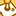# QlikView App Dev

Discussion Board for collaboration related to QlikView App Development.

Announcements
QlikWorld 2023, a live, in-person thrill ride. Save \$300 before February 6: REGISTER NOW!
cancel
Showing results for
Did you mean:Contributor III

## Weekstart in set analysis

Hi, QlikView

I have a dataset that looks like that

 Company Date Income Other Income Benz 02.01.2017 2.078.087 0 Benz 03.01.2017 3.560.614 5.000 Benz 04.01.2017 3.703.275 2.000 Benz 05.01.2017 3.524.276 1.000.000

I have one problem that I am trying to find solution. I have made a chart in the qlikview example.

That chart has two expressions sum (Income) and =sum({\$<Date={'>=\$(=WeekStart(Today(), 0))<=\$(=Today())'}>}[Other Income])

I wanted to only get the other income for the current week.

My problem is that when I click on a date like 23.1.2017 my chart looks like below (chart 2). I know the set analysis for Other Income is the reason behind this. But can I use another set analysis that will show other income equals zero when I click on 23.1.2017 like chart 2.

Any ideas

Chart 1

 Company Date Income Other Income Benz 23.1.2017 2.325.132 21.142 Benz 24.1.2017 0 32.563

Chart 2

 Company Date Income Other Income Benz 23.1.2017 2.325.132 21.142

Regards Darri

1 Solution

Accepted SolutionsChampion III

You can change the expression to below

=if(getselectedcount(Date)>0,sum([Other Income]),sum({\$<Date={'>=\$(=WeekStart(Today(), 0))<=\$(=Today())'}>}[Other Income]))

Vineeth Pujari
If a post helps to resolve your issue, please accept it as a Solution.
3 RepliesChampion III

You can change the expression to below

=if(getselectedcount(Date)>0,sum([Other Income]),sum({\$<Date={'>=\$(=WeekStart(Today(), 0))<=\$(=Today())'}>}[Other Income]))

Vineeth Pujari
If a post helps to resolve your issue, please accept it as a Solution.Contributor II

=if(getselectedcount(Date)>0,sum([Other Income]),sum({\$<Date={">=\$(=WeekStart(Today(), 0))<=\$(=Today())"}>}[Other Income]))Contributor II

You can try this expression to below

=if(getselectedcount(Date)>0,sum([Other Income]),sum({\$<Date={'>=\$(=WeekStart(Today(), 0))<=\$(=Today())'}>}[Other Income])) Unlinked ApkCommunity Browser